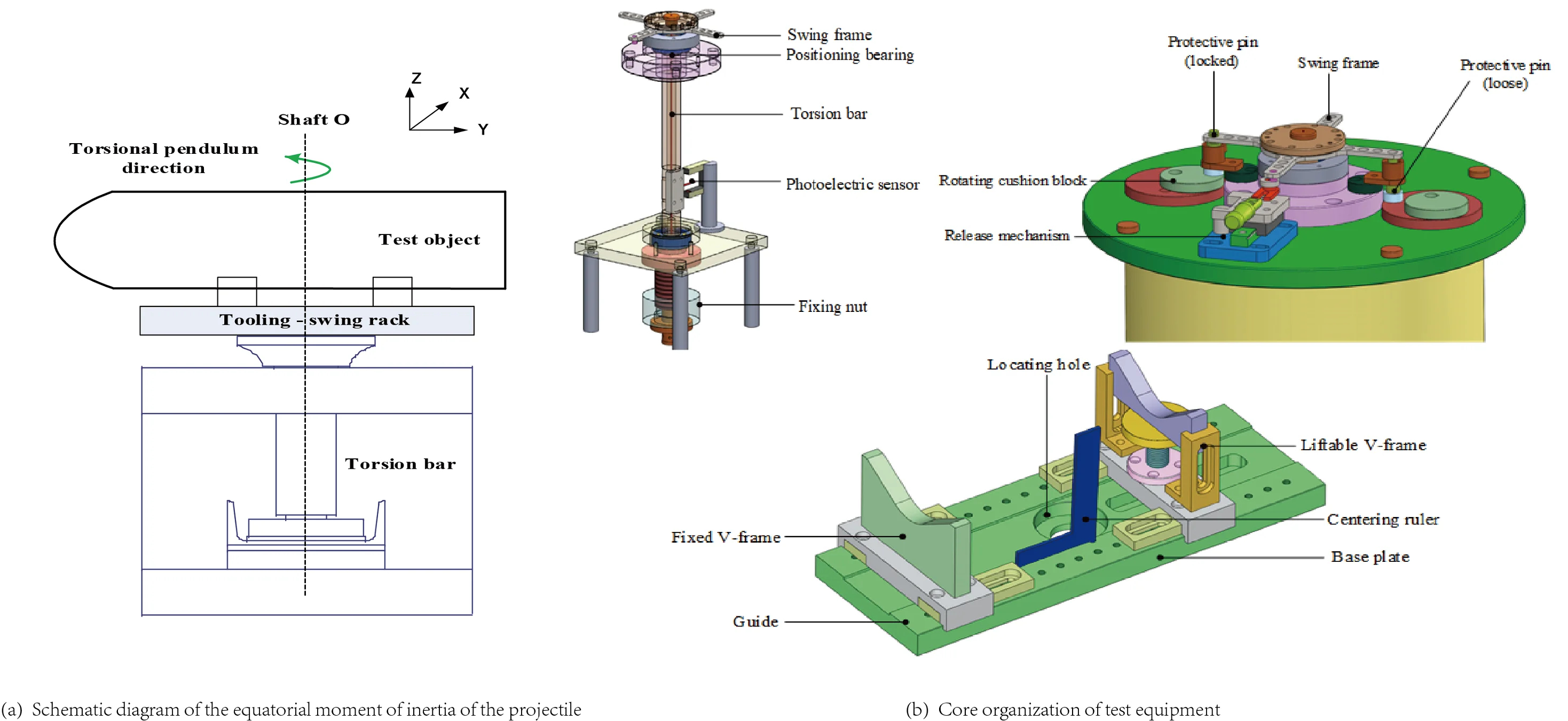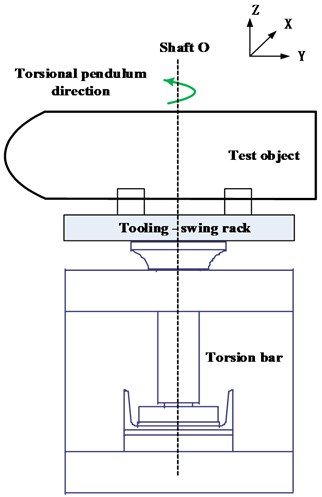Published: 13 June 2022

# Design and accuracy test of equatorial moment of inertia test equipment for projectile and rocket

Shukun Wang1
Jinpeng Mao2
Junye Li3
Liwei Sun4
Weihong Zhao5
Chunmin Shang6
1, 2, 3, 4, 5, 6Ministry of Education Key Laboratory for Cross-Scale Micro and Nano Manufacturing, Changchun University of Science and Technology, Changchun, China
Corresponding Author:
Junye Li
Views 160

#### Abstract

The moment of inertia is an important mass characteristic parameter. The precise measurement of the moment of inertia is a necessary step to study and control the flight orbit and attitude of missiles. To measure the equatorial moment of inertia of large projectiles, this paper uses the torsion pendulum method to measure the equatorial moment of inertia of large projectiles and deeply analyzes the measurement principle. The causes of measurement error are analyzed, and the measurement accuracy of the equipment is verified by experiments. The maximum relative error is less than 1 %, and the relative uncertainty is 1.3926 %, which meets the requirements of expected technical indicators.#### Highlights

• The test equipment of equatorial moment of inertia is designed
• The equatorial moment of inertia of a large projectile is measured based on the torsion pendulum method
• The causes of errors are analyzed

## 1. Introduction

When the projectile bypasses the center of mass and rotates in a plane perpendicular to the rotation axis, the measured moment of inertia is the equatorial moment of inertia. The commonly used methods to measure the moment of inertia mainly include the compound pendulum method , the torsion pendulum method , and the three-wire pendulum method . Che Ying and others designed and manufactured the instrument for measuring the moment of inertia of a small projectile  by using the double suspension wire torsion pendulum mechanism, which realizes the precise measurement of the moment of inertia of a small projectile, but the instrument is not suitable for the measurement of the moment of inertia of large projectile and arrow. Li Huipeng and others developed the missile moment of inertia test system based on the torsion pendulum method. Due to the use of an air-floating turntable mechanism, the measurement accuracy is less than 0.1% , but the manufacturing cost is high. Due to its advantages of good safety and high measurement accuracy, the torsion pendulum method has been more applied to the measurement of inertial parameters of high-tech equipment such as satellites  and missiles . In this paper, the torsion pendulum method is used to design the polar moment of inertia test equipment for projectile and arrow, and the accuracy of the equipment is analyzed, which can meet the needs of the equatorial moment of inertia test of projectile and arrow.

## 2. Principle of equatorial moment of inertia measurement

For projectiles with a large aspect ratio , the torsion pendulum method is mostly used to measure the equatorial moment of inertia. This method has the advantages of high accuracy and high test efficiency [9-11]. The principle of measuring the equatorial moment of inertia of large mass projectile and arrow by torsion pendulum method is shown in Fig. 1.

According to the law of rotation, the motion equation of the system is shown in Eq. (1):

1
$J\stackrel{¨}{\phi }+c\stackrel{˙}{\phi }+k\phi =0.$

Eq. (2) is a variation of Eq. (1):

2
$\stackrel{¨}{\phi }+2n\stackrel{˙}{\phi }+{p}^{2}\phi =0,$

where, $2n=c/J$ and ${p}^{2}=k/J$ are damping coefficient and undamped frequency respectively. When $n, the solution of the differential equation can be expressed as Eq. (3):

3
$\phi ={e}^{-nt}\left({c}_{1}\mathrm{c}\mathrm{o}\mathrm{s}\overline{p}t+{c}_{2}\mathrm{s}\mathrm{i}\mathrm{n}\overline{p}t\right),$

where: $\overline{p}=\sqrt{{p}^{2}-{n}^{2}}$, $\overline{T}=2\pi /\overline{p}=2\pi /\sqrt{{p}^{2}-{n}^{2}}$. Then the moment of inertia $J$ of the projectile is calculated by measuring the damping swing period $\overline{T}$.

Fig. 1Schematic diagram of the equatorial moment of inertia of the projectile## 3. Composition of measuring equipment

The equatorial moment of inertia measuring platform for projectile and rocket is composed of a base, torsion pendulum system, pendulum system, and test tooling. The core mechanism of the test equipment is shown in Fig. 2.

When measuring the equatorial moment of inertia, place the projectile and arrow horizontally on the tooling. After the system is stable, apply an external force and push the release handle reversely. The swing frame will twist freely. After swinging for a certain number of cycles, the computer will get the cycle value. Using the mathematical calculation model of an equatorial moment of inertia, the data are analyzed and processed by a computer, and then the equatorial moment of inertia to be measured is obtained.

## 4. Error analysis

### 4.1. Measurement error caused by damping

This measuring device belongs to an underdamped system. $n<1$, $\overline{p}=p\sqrt{1-\left({n}^{2}/{p}^{2}\right)}$, $\overline{T}=2\pi /\overline{p}=T/\sqrt{1-\left({n}^{2}/{p}^{2}\right)}$. It can be seen that the existence of damping causes the vibration period of the system to become larger. When ${n}^{2}/{p}^{2}=$ 0.05, the actual vibration period can be expressed as Eq. (4):

4
$\overline{T}=T\frac{1}{\sqrt{1-\left({n}^{2}/{p}^{2}\right)}}=1.001T.$

Therefore, the relative error of equatorial moment of inertia caused by damping can be expressed as Eq. (5):

5
$\mathrm{\Delta }=\frac{\overline{T}-T}{T}×100%=0.2%.$

Fig. 2Core organization of test equipment### 4.2. Measurement error caused by periodic measurement

The influence of periodic measurement error on the measurement of moment of inertia can be obtained from the error transfer function formula:

6
$\eta =\frac{\delta J}{J}=\frac{2AT\delta T}{A{T}^{2}}=2\frac{\delta T}{T}=0.05%.$

### 4.3. Measurement error caused by centroid position

As shown in Fig. 3, the error is caused by the non-coincidence between the ideal rotation axis and the actual rotation axis. According to the parallel axis theorem:

7
${J}_{{y}_{2}}={J}_{{y}_{1}}+M{d}^{2}.$

### 4.4. Analysis of experimental data

The standard sample is used to measure the equatorial moment of inertia. The experimental data are shown in Table 1. The basic Bessel formula  is as follows:

8
$s=\sqrt{\frac{\sum _{1}^{n}{v}_{i}^{2}}{n-1}},$

where ${v}_{i}$ represents the residual of the $i$-th measurement data.

Table 1Measurement results of equatorial moment of inertia of standard sample

 Number of experiments Standard value / kg⋅m2 Measurements / kg⋅m2 Relative error /$\mathrm{%}$ 1 102.28 102.65 0.3618 2 102.59 0.3031 3 103.12 0.8213 4 102.78 0.4889 5 102.82 0.5280 6 102.52 0.2347 7 102.66 0.3715 8 102.70 0.4106 9 102.54 0.2542 10 102.60 0.3129

According to Eq. (8), the standard uncertainty of equatorial moment of inertia measurement is $s=$ 0.4748 kg⋅m2, the expanded uncertainty is ${s}_{k}=$ 1.4244 kg⋅m2, and the relative uncertainty is 1.3926 %, which meets the accuracy requirements. The experimental results show that the maximum relative error of the equatorial moment of inertia of the standard sample is 0.8213 %, which meets the requirement that the relative error of the expected technical indicators is less than 1 %.

## 5. Conclusions

In this paper, the torsional pendulum method is used to measure the equatorial moment of inertia of large missiles and arrows, and the measurement principle is deeply analyzed. Based on the torsional pendulum method, an equatorial moment of inertia test equipment is designed. The equipment has the advantages of simple design and manufacture, safe and efficient structure, and can effectively measure the equatorial moment of inertia of large missiles and arrows. The causes of measurement error are analyzed, and the measurement accuracy of the equipment is verified by experiments. The maximum relative error is less than 1 %, and the relative uncertainty is 1.3926 %, which meets the requirements of expected technical indicators.

#### Acknowledgements

The authors would like to thank the national natural science foundation of China U1937201, Science and technology development plan project of Jilin province No. 20200301040RQ and 20210201057GX, Science and technology research project of Jilin Provincial Department of Education No. JJKH20220734KJ.